Courses

# JEE(MAIN) Mathematics Mock Test - 6

## 30 Questions MCQ Test JEE Main Mock Test Series 2020 & Previous Year Papers | JEE(MAIN) Mathematics Mock Test - 6

Description
This mock test of JEE(MAIN) Mathematics Mock Test - 6 for JEE helps you for every JEE entrance exam. This contains 30 Multiple Choice Questions for JEE JEE(MAIN) Mathematics Mock Test - 6 (mcq) to study with solutions a complete question bank. The solved questions answers in this JEE(MAIN) Mathematics Mock Test - 6 quiz give you a good mix of easy questions and tough questions. JEE students definitely take this JEE(MAIN) Mathematics Mock Test - 6 exercise for a better result in the exam. You can find other JEE(MAIN) Mathematics Mock Test - 6 extra questions, long questions & short questions for JEE on EduRev as well by searching above.
QUESTION: 1

Solution:
QUESTION: 2

Solution:
QUESTION: 3

### The general solution of the equation x2(dy/dx)=2 is

Solution:
QUESTION: 4

The area of the region bounded the curve y = 2x - x2 and the line y = x is

Solution:
QUESTION: 5

If line y=2x is a chord of the circle x2+y2-10x=0, the equation of the circle drawn on the chord assuming it as a diameter is

Solution:
QUESTION: 6

If (cos θ + i sin θ)(cos 2θ + i sin 2θ).....(cos mθ + i + sin mθ) = 1 then the value of θ ,is (m is an integer)

Solution: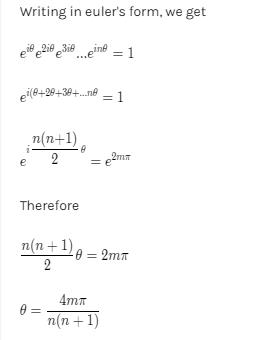QUESTION: 7

If circles x2+y2+2ax+c=0 and x2+y2+2by+c=0 touch each other, then

Solution:
QUESTION: 8

If the amplitude of z − 2 − 3i is π/4 , then the locus of z = x + iy is

Solution:
QUESTION: 9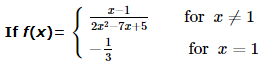then f ′ (1)  =

Solution:
QUESTION: 10

The negation of the statement ' he is rich and happy' is given by

Solution:
QUESTION: 11

If N N + denotes the set of all positive integers and if f : NN + → N is defined by f (n) = the sum of positive divisors of n then f (2 k . 3), where k is a positive integer is

Solution:

f(2k. 3) = The sum of positive divisors of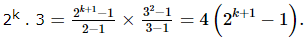QUESTION: 12

In the following question, a Statement of Assertion (A) is given followed by a corresponding Reason (R) just below it. Read the Statements carefully and mark the correct answer-
Assertion(A): The function f(x)=sin x is symmetric about the line x = 0
Reason (R): Every even function is symmetric about y-axis

Solution:
QUESTION: 13
In the following question, a Statement of Assertion (A) is given followed by a corresponding Reason (R) just below it. Read the Statements carefully and mark the correct answer-
Assertion (A): If n is a positive integer then 32n + 7 is divisible by 8
Reason (R): G.C.F. of 16 and 88 is 8
Solution:
QUESTION: 14

If 3, -2, are the eigen values of a non-singular matrix A and |A| = 4, then the eigen values of adj A are

Solution:
QUESTION: 15

The combined equation to the tangents to the parabola y2 = 4ax from an external point A (x1, y1) is

Solution:
QUESTION: 16

In throwing of two dice, the probability of getting a multiple of 4 is

Solution:
QUESTION: 17

If nPr=840, nCr=35, then n=

Solution: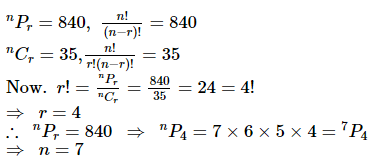QUESTION: 18

Mode is approximately given by

Solution:
QUESTION: 19

The equation of line passing through intersection point of lines x+5y+7=0 and 3x+2y-5=0 and perpendicular to line 7x+2y-5=0 is

Solution:
QUESTION: 20
The subnormal to the curve xy = c2 at any point varies directly as
Solution:
QUESTION: 21

If the direction cosines of a line are < (1/c), (1/c), (1/c) >, then

Solution:
QUESTION: 22

[(cos12°-sin12°)/(cos12°+sin12°]+[(sin147°)/(cos147°)]=

Solution:
QUESTION: 23

Three forces i+2j-3k, 2i+3j+4k and i-j+k are acting at a point (0,1,2). The modulus of moment of forces about the point (1,-2,0) is

Solution:
QUESTION: 24

The projection of the vector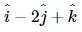on the vector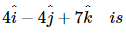Solution:
QUESTION: 25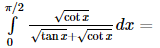Solution:
QUESTION: 26

If f : ℝ → ℝ is defined by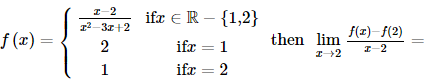Solution:
QUESTION: 27

In the following question, a Statement of Assertion (A) is given followed by a corresponding Reason (R) just below it. Read the Statements carefully and mark the correct answer-
Let f(x) = 2 + cos x for all real x.
Assertion(A): For each real t , there exists a point c in [ t,t + π ] such that f'(c) = 0.
Reason(R):f(t) = f(t + 2π) for each real t.

Solution:
QUESTION: 28

If x ∈ [-1, 1] then the minimum value of f(x) = x2 + x + 1 is

Solution: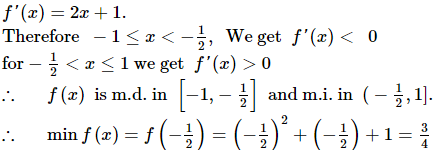QUESTION: 29

If -1, 0 and 1 are the elements of two matrices A and B of order 1 x 3 and of order 3 x 1 respectively, then A B equals -

Solution: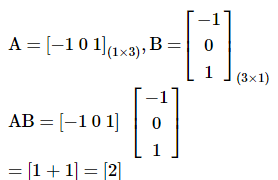QUESTION: 30

If the sum to infinity of the series 1 + 4 x + 7x2 + 10x3 + … is 35/16 , then the value of x is

Solution: